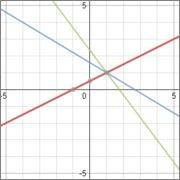# Understanding the meaning of Unique Solution

22990atinesh
General definition of System of Linear Equations says that

"If The system has a unique solution, It has independent set of Equations"​

Consider the system of linear equations
##x-2y=-1##
##3x+5y=8##
##4x+3y=7##
As we can see from the below graph that all the 3 line intersect at a single point ##\implies## System has a unique solution. But at the same time system is not independent as any equation can be derived from the algebraic manipulations of other two equations. So, how definition is true.General definition of System of Linear Equations says that

"If The system has a unique solution, It has independent set of Equations"​

So, how definition is true.

First, we should deal with the fact that "definitions" cannot be "false". A "conjecture" or "statement" may be true or false. A definition is merely information about how to interpret an undefined phrase in terms of statements that are already defined. So the "definition" of a "system" (of linear equations) should say that it is a set of linear equations in several unknowns. A "solution" to a "system" should also be defined. "Independence" of linear equations must also be defined.

The assertion that "if a system has a unique solution then the equations in it must be independent" is not a definition. It is a statement about things that are already defined. As you showed, it is a false statement. The correct statement is "If a system of linear equations is independent and there are as many equations as unknowns then the system has a unique solution."

It is possible to make definitions that are nonsensical in some way. For example, they might define things that do not exist. If you say "Biggy is defined to be a real number B such that for each real number x, B > x" then you have made a definition. The thing that is defined does not exist. However the definition is not a "false" statement. The statement that "There exists a real number equal to Biggy" is false. That statement is not a definition.

Mentor
General definition of System of Linear Equations says that

"If The system has a unique solution, It has independent set of Equations"​

Consider the system of linear equations
##x-2y=-1##
##3x+5y=8##
##4x+3y=7##
As we can see from the below graph that all the 3 line intersect at a single point ##\implies## System has a unique solution. But at the same time system is not independent as any equation can be derived from the algebraic manipulations of other two equations. So, how definition is true.This question is related to the other one you posted earlier. I'm not sure what your textbook means by "independent set of equations." The concepts of linear independence and linear dependence are usually found in the context of vectors or functions, rather than equations themselves. You should post your book's definition of "independent set of equations."

In any case, the general definition you cite above is not true. Since the third equation can be obtained by adding the first and second equations, the system appears to me to consist of a set of dependent equations. The system does have a unique solution, but also is made up of what appear to be dependent equations.

•